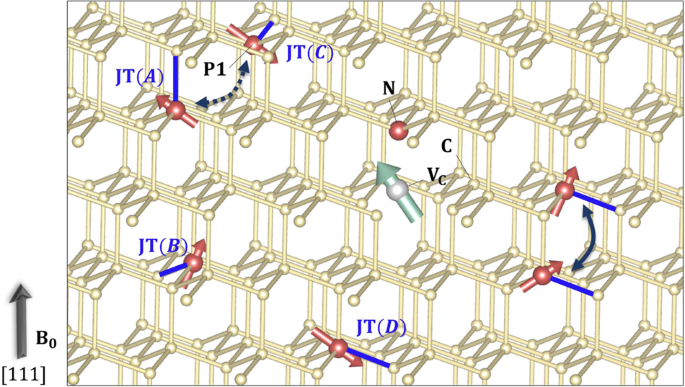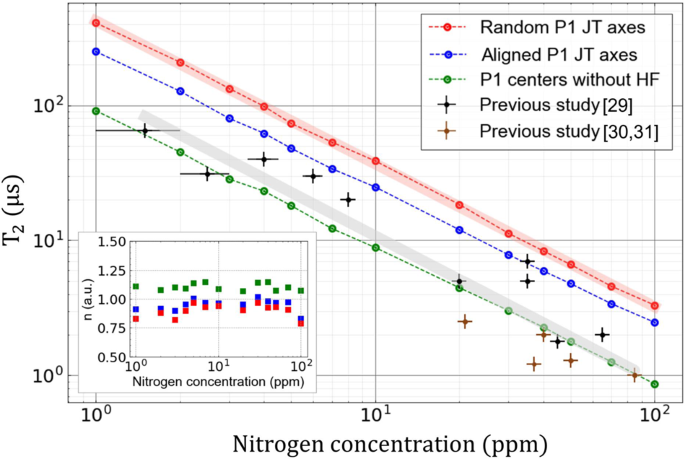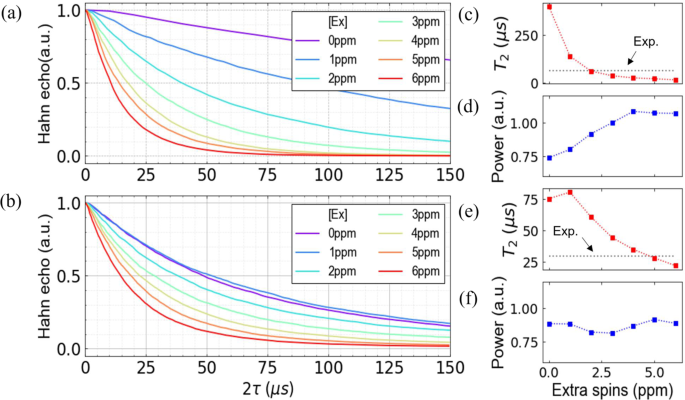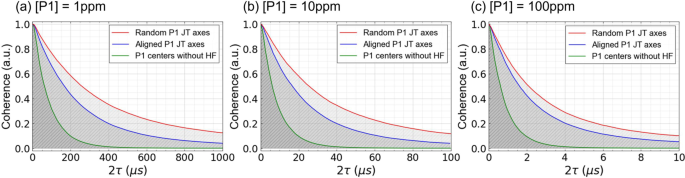## Introduction

Nitrogen-vacancy (NV) centers in diamond are one of the leading solid-state-based qubit platforms, enabling diverse quantum applications including quantum information processing1,2,3,4, quantum sensing5,6,7,8, and quantum networks9,10. For a spin qubit, a long spin lifetime is one of the key properties that an NV center should possess because it plays a critical role in determining the performance of a spin qubit in quantum applications6. Combined with spin bath decoupling methods or bath engineering techniques, the Hahn-echo spin-coherence time (T2) of an NV center has been shown to reach ~1 s11,12,13. In practical applications, however, the spin lifetime of an NV center could be significantly degraded due to intensified decoherence caused by paramagnetic spin defects14, which in turn could limit the range of NV-spin qubit-based quantum applications14,15.

NV-spin decoherence has been extensively investigated and the dominant mechanism has been found to involve the coupling of the NV-spin dipolar to the fluctuating spin bath in diamond16. Two dominant types of spin baths identified are those with 13C nuclear spins (natural abundance 1.1%, I = 1/2) and those considered as electronic spin baths with substitutional nitrogen electron-nuclear spins (the so-called P1 bath, 14N 99.6%, S = 1/2, and I = 1). In a 1.1% 13C nuclear-spin-dominant bath, the NV-spin’s Hahn-echo coherence time (T2) has been reported to be larger than 600 μs17,18,19 and its decoherence dynamics is theoretically well understood20. Building upon this established knowledge, several methods have also been developed to suppress the 13C-induced decoherence of the NV spin, including a dynamical decoupling method21 and 12C isotopic purification of a diamond sample18. Meanwhile, it is well known that the paramagnetic spins in diamond, or P1 centers22, are one of the dominant sources of NV-spin decoherence23,24,25,26,27. It has been shown that the NV-spin lifetime is much more fragile under the presence of P1 spins than in a 13C nuclear-spin bath owing to the larger gyromagnetic ratio of P1 spins24,25,27,28. Nevertheless, P1-induced NV-spin decoherence is less understood than 13C-induced decoherence.

Recently, understanding the NV-spin decoherence mechanism due to various P1 concentrations ([P1]) has gained much interest29,30,31,32,33,34 in several important applications such as nuclear-spin hyperpolarization35, quantum sensing5,6, and quantum information applications1,36,37. Such applications may require either high NV densities or the controlled creation of P1 centers. In the NV center generation process, however, P1 centers are inevitably created with different concentrations (1–100 ppm) owing to the low N-to-NV center yield rate (<25%)35,38, which, in turn, may lead to the degraded coherence of the NV center due to the P1 centers7. Thus, it is of paramount importance to systematically understand P1-induced NV decoherence as a function of [P1].

Recent studies have made significant progress towards the quantitative analysis of P1-induced NV-spin decoherence. In particular, it has become possible to determine [P1] in a diamond sample with high precision30,31, enabling measurements of the NV’s T2 as a function of [P1]29,30,31,32. Recently, Bauch et al.29 reported the T2 of an NV-spin ensemble as a function of [P1], showing a linear dependence of T2 on [P1] on a log scale with a stretched exponential parameter of ~1.37. In another recent work by Li et al.30 a local spin bath measurement technique is developed to extract the position-dependent concentration of various paramagnetic defects in a diamond sample. Importantly, however, the linear dependence between T2 and [P1] found in the semi-classical simulation of the previous study29 was much less clear. In addition, the stretching exponent measured varied significantly depending on [P1], which also deviates from that of the previous result29. Li et al.30 and other researchers have suggested that the deviation may be caused by extra paramagnetic defects other than the P1 centers in the sample.

The lack of a full understanding of the NV’s T2 vs. [P1] and the role of extra electronic spins can benefit from the accurate theoretical prediction of T2([P1]), which can provide an upper bound for T2 and a reference for the stretched exponential parameter of a P1-dominant bath. Early theoretical studies have shown that the basic property of P1-induced NV-spin decoherence can be analyzed by using semi-classical theories based on the Ornstein–Uhlenbeck (O–U) process. Notably, a similar semi-classical method was used to understand recent experimental measurements29,30, and the overall qualitative tendency of T2 as a function of [P1] was reproduced. The semi-classical results, however, underestimate the T2 and overestimate the slope over a wide range of P1 concentrations29. In this regard, first-principles calculations with a predictive power are necessary to provide a quantitative theoretical guidance to P1-induced NV decoherence.

In this study, we perform fully quantum mechanical calculations by combining cluster correlation expansion and density functional theory (DFT) to investigate the P1-induced decoherence dynamics of a negatively charged NV ensemble for a broad range of [P1]. We compute the Hahn-echo T2 as a function of [P1] and our results provide a theoretical upper bound for T2 from 1 to 100 ppm. We show that the T2([P1]) exhibits a clear linear dependence on a log scale with a slope of −1.06, which is in excellent agreement with the experimental result of −1.0729,30,31. We found that the stretched exponential parameter is close to 1 for the [P1] from 1 to 100 ppm. Building upon these results, we show that the Jahn–Teller-derived anisotropy in the hyperfine interaction of the P1 center plays a crucial role in determining P1 bath dynamics. Our study offers a complete understanding of the P1-induced spin decoherence dynamics of an NV ensemble, which can provide a key reference for developing diamond samples with optimized NV-spin lifetimes for different applications.

## Results

### System and model

We adopt a central spin model to compute the decoherence dynamics of an NV-spin interacting with a substitutional nitrogen impurity spin bath. We use the Hahn-echo pulse sequence, which has a π pulse in between two free evolution time periods τ to compute the homogeneous dephasing time T2 of NV-spin ensembles39,40. The degree of NV-spin decoherence is obtained by computing the off-diagonal element of the reduced density matrix of the NV spin after tracing out the bath degrees of freedom at the end of the 2τ free evolution time39 (see Methods for details).

Figure 1 shows our central spin model in detail. The NV center is a spin-1 electronic defect, which consists of a C vacancy and substitutional N atom paired along the  crystal direction. An external magnetic field of 500 G is applied in the same direction as the symmetry axis of the NV spin. The P1 center is an isolated substitutional nitrogen impurity that replaces a carbon atom in the diamond lattice. In our model, P1 spins are randomly distributed around the NV spins, and we consider various [P1] from 1 to 100 ppm. An NV spin is coupled to the P1 centers via the magnetic dipole–dipole interaction (see Supplementary Note 1). The P1 center accompanies an electron spin-1/2 and a nuclear spins-1 (spin-1/2) for 14N (15N, natural abundance of 0.37%), which are strongly coupled to each other via a hyperfine interaction (see Supplementary Note 2). The electronic spin of the P1 center is highly localized at the lattice site due to the Jahn–Teller (JT) effect41.Fig. 1: Diamond NV center in a P1 bath.

The JT effect creates strong anisotropy in the hyperfine interaction of the P1 center42,43 and induces a strong lattice relaxation in such a way that the N atom is distorted away from one of the four nearest neighboring C atoms, leading to four possible hyperfine anisotropy axes. In Supplementary Information, we provide a detailed description of the anisotropic hyperfine tensors. We compared our DFT calculations with experimental results37, the comparison of which demonstrated excellent agreement for the four different hyperfine anisotropy axes (see Supplementary Note 2). In our central spin model (see Fig. 1), the four possible anisotropy axes are randomly assigned to the P1 centers. In addition, the given anisotropy axis for the P1 centers is fixed during the simulation as the time scale of the JT axis change is known to be from 103 to 105 s in low temperatures (≤200 K)44, which is much larger than the time scale of NV-spin decoherence, which is in the order of hundreds of microseconds.

To compute the NV-spin’s T2 induced by a large number of P1 centers, we employ the cluster correlation expansion method (CCE)39,40,45, which enables a predictive quantum mechanical computation of T2 without assuming any adjustable theoretical parameters. The CCE method was successfully applied to a wide range of central spin models including both nuclear20,46,47 and electron spin baths48,49, yielding an excellent agreement with experimental results47,49. Further details on the spin Hamiltonian, theoretical methods, and the numerical convergence tests can be found in the Methods section and Supplementary Notes3 and 4.

### The coherence time of the NV center as a function of [P1]

Figure 2 presents the computed Hahn-echo T2 of NV ensembles in a P1 bath as a function of [P1] from 1 to 100 ppm. The computed T2 time was compared with previous experimental results29,30,31. Our findings indicate that the computed T2 time provides a proper upper bound for the spin-coherence time of the NV ensemble in the P1 environment. In Fig. 2, it can be observed that the theoretical results are consistently 2–4 times larger than the experimental results: the theoretical (experimental) T2 times are 98.2 μs (40 μs), 18.4 μs (5 μs), and 8.4 μs (2 μs) at 4 ppm, 20 ppm, and 40 ppm, respectively. Furthermore, the theoretical T2 time shows a clear linear dependence on [P1] on a log scale with a slope of −1.06, which is in excellent agreement with the previous experimental observations29,30,31 that present a slope of −1.07 on a log scale.Fig. 2: Coherence times of diamond NV ensembles in a P1 bath.

In terms of the stretching exponent (n) of the NV-spin’s coherence decay curve, n increases slowly as a function of [P1] and the average value is found to be 0.9 as shown in Fig. 2. The n values are ~0.8 and ~0.9 at low [P1] around 3 ppm and at high [P1] around 50 ppm, respectively. The CCE results are reasonably consistent with recent experimental measurements, which yielded $$n \,=\, 1.37 \,\pm\, 0.23$$29. It is worth noting that O–U based semi-classical theory predictions of n could vary from 1 (motional-narrowing regime) to 3 (quasi-static regime)29,33. Several early experimental studies reported scattered results for n from 1 to 326,31,33. For the NV-spin ensemble in a pure P1 bath, our CCE calculations show that the shape of the NV’s spin-echo decay curve is close to the curve of a single exponential function.

The large deviation of the experimental T2 results from the theoretical calculations obtained for a pure P1 bath indicates the important potential role of parasitic electron spins in addition to P1 centers that act as extra decoherence sources30,34,50. In Fig. 3, the effect of adding extra electronic spins (S = 1/2 with g = 2) in a P1 bath is examined and results indicate that the experimental T2 time can be reproduced in theory when the concentration of the extra spins ([Ex]) is similar to [P1]. Figure 3a, b show the computed NV’s spin coherence for [P1] = 1 ppm and 5 ppm, respectively, by varying [Ex] from 0 to 6 ppm. It can be observed that the NV’s spin coherence is highly sensitive to the presence of extra spins, and T2 and n significantly change as [Ex] increases. When [Ex] is 2 ppm (see Fig. 3c, d), the computed T2 for [P1] = 1 ppm is 60.7 μs, which matches well with the experimental T2 time of 65 μs. n is computed to be ~0.74 and ~0.92 for [Ex] = 0 ppm and 2 ppm, respectively. For a P1 spin bath with [P1] = 5 ppm (see Fig. 3e, f), the experimentally measured T2 time of ~30 μs is theoretically reproduced when [Ex] is 5 ppm, yielding T2 = 28.2 μs. The same trend holds for a P1 bath with [P1] = 12 ppm and [P1] = 50 ppm as shown in Supplementary Fig. 5. Our results suggest the importance of considering extra electronic spins and their potential correlation with the P1 centers to describe the NV-spin decoherence dynamics more accurately in a P1 bath.Fig. 3: NV decoherence in a P1 bath with extra electron spins.

### The role of 14N nuclear spin in the P1-P1 flip-flop dynamics

To better understand P1-induced NV-spin decoherence, the P1 bath dynamics were analyzed with a focus on the role of the N nuclear spin in controlling the flip-flop dynamics between the P1 electron spins. It is worth mentioning that the nuclear-spin flip-flop dynamics can be safely ignored because its time scale is much longer than those of the electron spin flip-flop transition rate and NV decoherence. With the electron spin flip-flop transition as the dominant source of magnetic field noise from the bath, fast flip-flop transitions imply fast NV-spin coherence decays. In what follows, we show that the P1 electron spin flip-flop transitions are significantly suppressed by the hyperfine interaction of the P1 center and JT-derived anisotropy in the hyperfine interaction.

Figure 4 compares the NV-spin coherence computed for a pure P1 bath, whose JT axes are random, with two hypothetical P1 bath models for [P1] = 1, 10, and 100 ppm: the P1 centers with no-hyperfine interaction and the P1 centers whose JT axes are all fixed in one direction. The P1 spin bath with no-hyperfine interaction is effectively the same as a bare electron spin bath in the time scale of a few hundreds of microseconds because the slow N nuclear-spin bath is decoupled from the electron spins. As shown in Fig. 4, the NV coherence in such “no-hyperfine” P1 bath model quickly decays due to the flip-flop transitions between the bare electron spins, yielding T2 times of 91.15 μs, 8.85 μs, and 0.87 μs for [P1] = 1, 10, and 100 ppm, respectively. Interestingly, however, we observed that the NV’s coherence is significantly enhanced when we include the hyperfine interaction between the electron and nuclear spins of the P1 center. For [P1] = 1, 10, and 100 ppm, the T2 times are increased to 249.62 μs, 24.83 μs, and 2.47 μs, respectively, when the JT axes are fixed to one direction. The T2 times are further increased to 406.61 μs, 38.73 μs, and 3.32 μs for [P1] = 1, 10, and 100 ppm, respectively, when the JT axes in the P1 bath are randomized. In addition to the P1 concentrations considered in Fig. 4, we also compared the T2 times computed using the three bath models for other [P1] from 1 ppm to 100 ppm, the results of which are reported in Fig. 2 and from which the same conclusions are drawn. Our calculation results show that the electron spin flip-flop dynamics are significantly suppressed in the presence of the N nuclear spin due to the hyperfine interaction and JT-induced anisotropy in the hyperfine interaction.Fig. 4: Spin coherence of NV ensembles in a P1 bath.

To elucidate the hyperfine-induced suppression of the P1 electron spin flip-flop dynamics, we analyzed the energy levels of a P1-P1 spin pair for the three P1 bath models in Fig. 5. Figure 5a shows the energy levels in the absence of the hyperfine interaction. We only considered the states whose electron spins are up and down ($$\left|\uparrow\downarrow\rangle\right. \;or\; \left|\downarrow \uparrow\rangle\right.$$) because they are coupled by the electron spin dipolar flip-flop interaction. Considering the nuclear-spin state ($$\left| +\rangle \right.,\left| 0\rangle \right.,\left| -\rangle \right.$$), there are 18 possible states whose electron spins are up and down as listed in Fig. 5b. In the absence of the hyperfine interaction, there are 9 active flip-flop transition channels as shown in Fig. 5a due to the nuclear Zeeman energy splitting ($$\Delta _n\,\sim\,$$ 154 kHz) and the mismatch in the nuclear-spin states (i.e., $$\left| { \uparrow \,+\!\downarrow 0} \rangle\right. \,\leftrightarrow\, \left| {\downarrow \,+\!\uparrow 0} \rangle\right.$$ is allowed, $$\left| {\uparrow \,-\!\downarrow -} \rangle\right. \,\leftrightarrow\, \left| {\downarrow 0 \uparrow - } \rangle\right.$$ is not allowed). However, when the hyperfine interaction is turned on in the bath, the number of these flip-flop channels is greatly reduced. For two P1 centers having the same JT axes, the number of flip-flop channels is three due to the energy level mismatch induced by the hyperfine interaction ($$\Delta _{{{{\mathrm{HF}}}}} \,\approx\, \frac{1}{2}{{{\mathrm{A}}}}_{||}^{{{{\mathrm{on}}}}} \,\approx\, 57\;{{{\mathrm{MHz}}}}$$). In addition, for a P1-P1 pair with different JT axes, the degenerated states obtain an additional energy shift ($${{{\mathrm{\delta }}}}_{{{{\mathrm{HF}}}}} \,\approx\, {{{\mathrm{A}}}}_\parallel \,-\, {{{\mathrm{A}}}}_\parallel ^\prime \,\approx\, 29\,{{{\mathrm{MHz}}}}$$), which reduces the number of flip-flop channels to only one. Our results show that the electron spin flip-flop transitions are largely suppressed in the presence of the JT-derived anisotropic hyperfine interaction.Fig. 5: Energy levels of a P1-P1 pair.

## Discussion

We performed full quantum calculations of the T2 time of diamond NV-spin ensembles in a P1 spin bath with the function of the P1 concentration varying from 1 ppm to 100 ppm by combining DFT and cluster correlation expansion. Our results show a clear linear dependence of the T2 time on [P1] on a log scale with a slope of −1.07. By systematically analyzing three different P1 spin bath models, we have found that the electron spin flip-flop dynamics in a P1 bath are largely suppressed in the presence of the anisotropic P1 hyperfine interaction. It is shown that the N nuclear spin induces various shifts in the P1 energy levels on the order of hundreds of MHz through hyperfine coupling, suppressing the electron spin flip-flop transitions.

Additionally, the computed T2 times are consistently larger than previous experimental results, which indicates the importance of other decoherence sources in describing the NV-spin decoherence in a P1 spin bath. After implementing extra electron spins as an additional source of decoherence, we found that the calculated T2 time becomes similar to the experimental T2 time when the concentration of the extra spins is similar to the P1 concentration. It should be noted, however, that other paramagnetic defects or complexes may be generated in different diamond sample preparation conditions, acting as extra parasitic spins in the diamond lattice. Further systematic investigation on other paramagnetic spins will help to better understand NV-spin decoherence in practical applications.

Electron dipolar spin ensembles in diamond are already being exploited for practical applications such as quantum sensing or quantum simulations. Understanding the bath spin ensemble dynamics derived from the results obtained in our study could be a vital resource in the engineering of NV-spin-based scalable quantum systems for quantum-enhanced technologies.

## Methods

### Cluster correlation expansion computation

To compute the T2 time of diamond NV ensembles, we implemented the single-sample CCE theory in our in-house CCE code, and several important features of our CCE computation can be summarized as follows. The P1 bath was clusterized in terms of the number of P1 centers instead of the number of spins in the original CCE formulation. For example, in our CCE-2 method, two P1 centers are considered in a cluster. In this way, we treat both the electron spin and the 14N nuclear spin on an equal footing and fully consider the anisotropy of the hyperfine tensor for individual JT axes. In addition, we employed the so-called single-sample approach, which treats each bath state independently39. We found that our single-sample CCE calculations give a converged T2 time when averaged over 100 bath states. For an ensemble average, we used 100 different bath spin configurations. We note that in the single-sample CCE method, one can take into account the interaction between clustered bath spins and external spins outside the cluster by adding their Ising-like interaction as a mean-field effect. The single-sample CCE method combined with the mean-field method was shown to produce a robust solution for sparse electron spin baths at the CCE-2 level of theory48,49, which we adopted in this study. In Supplementary Note 2, details of our CCE computation and convergence test results are provided.

### Density functional theory calculations

To compute the spin Hamiltonian parameters of a P1 center, we conducted DFT calculations using a plane-wave basis set with an energy cutoff of 85 Ry along with projector-augmented wave (PAW) pseudopotentials (QE PAW v0.3.1 set)51,52 as implemented in the QUANTUM ESPRESSO code53. We employed the Perdew–Burke–Ernzerhof semi-local functional to describe the exchange-correlation potential54,55. To simulate the presence of an isolated P1 center in diamond, we used the supercell method. The supercell is oriented in the  direction and it contains 1007 atoms. The Brillouin zone is sampled with the Γ point only. We used the GIPAW module of the QE code to compute the hyperfine tensor and the quadrupole moment of a P1 center. The core polarization effects were included in the evaluation of spin densities near the nuclei. Our DFT calculations were in excellent agreement with the experimental results for the hyperfine tensor and the quadrupole moment as described in detail in Supplementary Note 2.